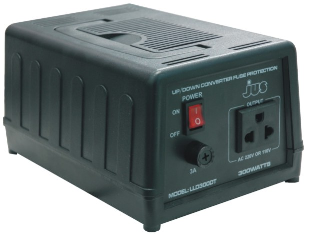﻿ What is a Step-down Transformer?

# What is a Step-down Transformer?A Step-down Transformer is an AC-AC converter which converts the input AC signal into an AC signal with a lower voltage.A step-down transformer acts as a voltage-decreasing device. The amount by which it decreases the input voltage depends on the ratio of the the number of turns in the primary coil to the number of turns in the secondary coil.

If, for example, the primary coil has double the amount of turns as the secondary coil, the ratio will be 2:1 and the output voltage will be half the input voltage.

Though step-down transformers decrease the voltage of the output voltage, the overall power is not decreased. Transformers are simply conversion devices. They do not create voltage or power. So if a step-down transformer decreases voltage, it increases current. If it cuts the voltage output in half, the current output doubles. So that the output signal now has double the current capability as the input signal. Step-down transformers never create or loser power; they only convert it into different forms.